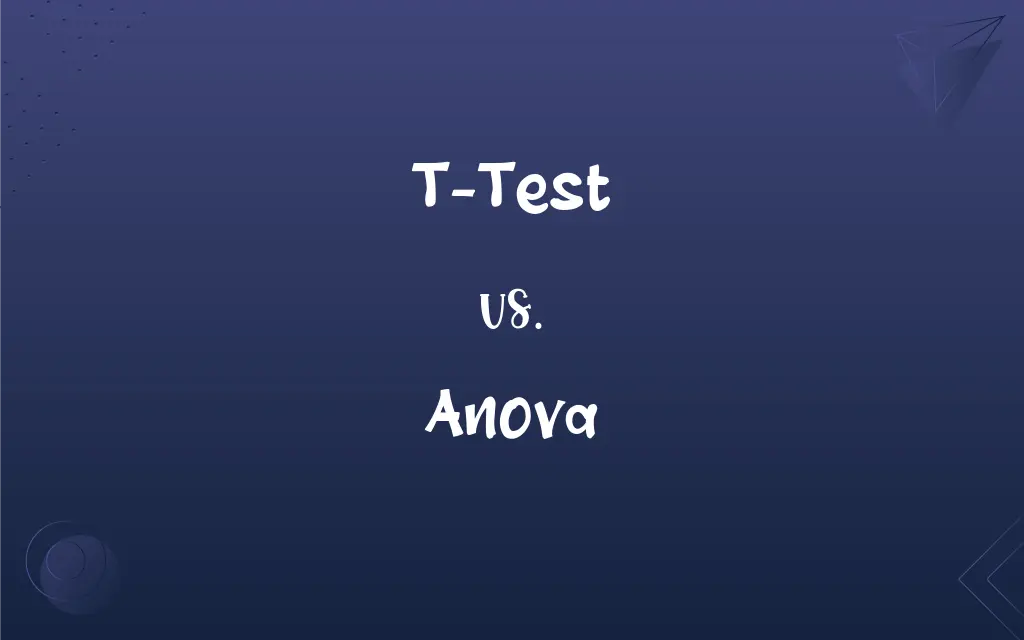# T-Test vs. ANOVA: What's the Difference?Edited by Huma Saeed || By Sumera Saeed || Updated on October 27, 2023
The t-test assesses differences between two groups, while ANOVA evaluates differences among three or more groups.## Key Differences

The t-test and ANOVA are both statistical methods used to determine if there are significant differences in group means. They are integral tools in experimental research, especially when comparing group averages.
While the t-test is employed to compare the means of two groups, ANOVA is used when there are more than two groups involved. If you're comparing the scores of two different teaching methods, you'd use a t-test. However, if there are three or more teaching methods, ANOVA would be the appropriate test.
The underlying assumptions for both t-test and ANOVA include the normality of the data and homogeneity of variances. However, when a significant difference is found in ANOVA, post hoc tests are conducted to determine which groups differ from each other, something not necessary with the t-test due to its binary nature.
The t-test provides a specific result about two group means, while ANOVA gives a general result about three or more group means. After obtaining a significant ANOVA result, further analyses are needed to pinpoint the specific groups with differing means. In contrast, the t-test directly indicates where the difference lies since there are only two groups.
In essence, both the t-test and ANOVA serve to compare group means, but they differ in the number of groups they analyze. It's critical to choose the right test based on the study design to ensure accurate results.

## Comparison Chart

### Number of Groups

Compares 2 groups.
Compares 3 or more groups.

### Result Interpretation

Directly shows which group differs.
Needs post hoc tests to determine specific group differences

### Test Statistic

Produces a t-value.
Produces an F-value.

### Assumptions

Normality, homogeneity of variances, independent samples.
Same, but more critical due to more groups.

### Use Case

Comparing two methods or treatments.
Comparing multiple methods or treatments.

## T-Test and ANOVA Definitions

#### T-Test

A statistical test comparing means of two groups.
The t-test revealed a significant difference between the control and experimental group.

#### Anova

A statistical method for assessing multiple group variances.
Using ANOVA, we found variability in scores among all age groups.

#### T-Test

A method determining if two datasets are significantly different.
Using the t-test, we found that method A was more effective than method B.

#### Anova

A tool to gauge significance among several experimental conditions.
ANOVA suggested that location, time, and method all affected the outcome.

#### T-Test

A test comparing the averages of two populations or samples.
The t-test showed that men and women had different average scores.

#### Anova

Analysis determining differences among multiple datasets.
ANOVA revealed that all three drugs had distinct effects.

#### T-Test

A two-group hypothesis testing procedure.
The t-test confirmed our hypothesis about the new drug's efficacy.

#### Anova

A test to compare means of three or more groups.
ANOVA indicated a significant difference among the four training methods.

#### T-Test

A tool to assess the significance of a binary experimental difference.
Through a t-test, we discerned a marked difference between the pre-test and post-test scores.

#### Anova

A technique to discern variability among multiple group averages.
Through ANOVA, we detected variations in performance across all teams.

## FAQs

#### What are the t-test and ANOVA used for?

The t-test compares means of two groups, while ANOVA compares means of three or more groups.

#### Are the assumptions for t-test and ANOVA similar?

Yes, they include normality, homogeneity of variances, and independent samples.

#### If ANOVA indicates a significant difference, which groups are different?

You'd need post hoc tests after ANOVA to determine specific group differences.

#### What's a two-way ANOVA?

It's an ANOVA variant analyzing the effects of two independent variables simultaneously.

#### What's the primary output of a t-test and ANOVA?

The t-test produces a t-value, while ANOVA produces an F-value.

#### What happens if I use a t-test for multiple pairwise comparisons?

This increases the chance of Type I error; ANOVA is recommended for multiple comparisons.

#### Are there one-sample versions for the t-test and ANOVA?

Yes, for the t-test. For ANOVA, it's inherently for comparing multiple groups.

#### Are t-test and ANOVA affected by outliers?

Yes, outliers can influence results; it's essential to check for them before analysis.

#### Why not always use ANOVA instead of the t-test?

ANOVA is more complex and requires post hoc tests for two groups, making the t-test more straightforward.

#### Can both t-test and ANOVA be used for non-normally distributed data?

Ideally no, but there are non-parametric alternatives available.

#### What type of data is suitable for t-test and ANOVA?

They both require interval or ratio-level data.

#### What is the paired t-test?

It's a t-test variant comparing means of the same group at different times or conditions.

#### How are effect sizes measured in t-test and ANOVA?

Commonly, Cohen's d for t-test and η^2 (eta squared) for ANOVA.

#### Do t-test and ANOVA require equal sample sizes?

No, but unequal sizes can affect power and the validity of assumptions.

#### Can I use a t-test for three groups?

No, for three or more groups, you should use ANOVA.

#### How do I interpret a significant t-test result?

It indicates a significant difference between the two group means.

#### What's a post hoc test in the context of ANOVA?

It's a follow-up test to determine which specific group pairs differ after a significant ANOVA result.

#### Can I perform a t-test and ANOVA on ranked data?

Non-parametric alternatives like Mann-Whitney U (for t-test) and Kruskal-Wallis (for ANOVA) are suitable for ranked data.

#### Can ANOVA compare more than just means?

Standard ANOVA compares means, but there are variants that compare other statistics.

#### How can I report t-test and ANOVA results?

Typically, you report the test statistic (t or F), degrees of freedom, and p-value.